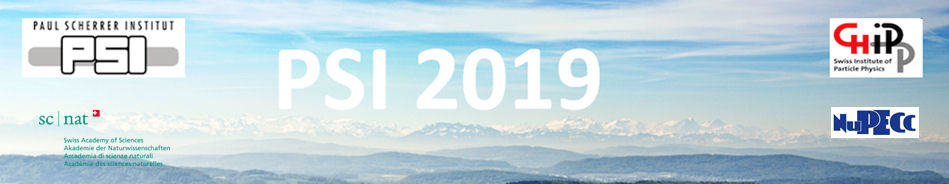#Physics of fundamental Symmetries and Interactions - PSI2019

Oct 20 – 25, 2019
PSI
Europe/Zurich timezone

## Nondecoupling Signatures of Supersymmetry and an $L_{\mu} - L_{\tau}$ Gauge Boson at Belle-II

Oct 22, 2019, 5:59 PM
1m
WHGA/001 - Auditorium (PSI)

Poster

### Speaker

Heerak Banerjee (Indian Association for the Cultivation of Science)

### Description

We propose that the $\gamma + \not{\hspace{-0.3em}E}$ signal at the Belle-II detector will be a smoking gun for supersymmetry (SUSY) in the presence of a gauged $U(1)_{L_{\mu}-L_{\tau}}$ symmetry. A striking consequence of breaking the enhanced symmetry appearing in the limit of degenerate (s)leptons is the nondecoupling of the radiative contribution of heavy charged sleptons to the $\gamma - Z^\prime$ kinetic mixing. The signal process, $e^+ e^- \rightarrow \gamma Z^\prime \rightarrow \gamma + \not{\hspace{-0.3em}E}$, is an outcome of this ubiquitous feature. We take into account the severe constraints on gauged $U(1)_{L_{\mu}-L_{\tau}}$ models by several low-energy observables and show that any significant excess in all but the highest photon energy bin would be an undeniable signature of such heavy scalar fields in SUSY coupling to $Z^\prime$. The number of signal events depends crucially on the logarithm of the ratio of stau to smuon mass in the presence of SUSY. In addition, the number is also inversely proportional to the $e^+-e^-$ collision energy, making a low-energy, high-luminosity collider like Belle-II an ideal testing ground for this channel. This process can probe large swathes of the slepton mass ratio vs the additional gauge coupling ($g_X$) parameter space. More importantly, it can explore the narrow slice of $M_{Z^{\prime}}-g_X$ parameter space still allowed in gauged $U(1)_{L_{\mu}-L_{\tau}}$ models for superheavy sparticles.

### Primary authors

Heerak Banerjee (Indian Association for the Cultivation of Science) Prof. Sourov Roy (Indian Association for the Cultivation of Science)